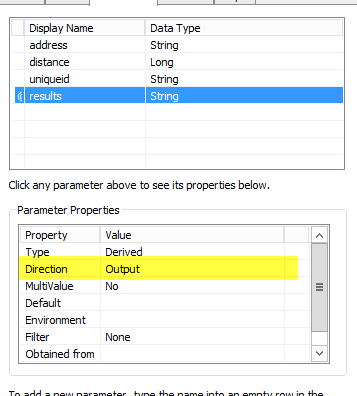# python to service

319
2
03-25-2020 06:39 AMFrequent Contributor

I have a simple example python script below that accepts an address and is trying to return the XY.

My plan is to take this python script and create a tool and publish this to ArcGIS Server as a service

I can then call the service and pass it the address parameter.  THe code will run and set a variable to the XY location of the geocoded address.  Great everything works....

How do I get that XY back to the user that is passing the address parameter?

``````import arcpy

# Geting user input for address

# SEARCH PARAMETERS FROM SERVICE
print ""

# GEOCODERS

##clean up the address for url encoding

lookup = requests.get(geoCodeUrl + "?SingleLine=" + address + "&outSR=" + outSR + "&maxLocations=1&f=pjson")
#get results to variable
data = lookup.json()

if data["candidates"]:
return data
else:

# CALL GEOCODER

# GET RESULTS
coordinateX = geocodeResult["candidates"]["location"]["x"]
coordinateY = geocodeResult["candidates"]["location"]["y"]
strcoordinateX = str(coordinateX)
strcoordinateY = str(coordinateY)

# SHOW RESULTS
print "gecode result: " + str(geocodeResult)
print "-------------------"
print "-------------------"
print "X coordinate: " + str(coordinateX)
print "-------------------"
print "Y coordinate: " + str(coordinateY)
print "-------------------"
‍‍‍‍‍‍‍‍‍‍‍‍‍‍‍‍‍‍‍‍‍‍‍‍‍‍‍‍‍‍‍‍‍‍‍‍‍‍‍‍‍‍‍‍‍‍‍``````
1 Solution

Accepted SolutionsFrequent Contributor

I set an output parameter in the python tool and then passed the value as such

I had 3 input parameters and set the 4th to an output parameter...dont forget the index starts at 0 so the 4th would be 3

``````msg =("testing result value")
resultMsg = msg
arcpy.SetParameterAsText(3, resultMsg)‍‍‍‍``````2 RepliesFrequent Contributor

Do I have to add an out parameter to the toolbox tool that is running the script?

if I have to do that what do I put in the python script to write the values to the output parameter?Frequent Contributor

I set an output parameter in the python tool and then passed the value as such

I had 3 input parameters and set the 4th to an output parameter...dont forget the index starts at 0 so the 4th would be 3

``````msg =("testing result value")